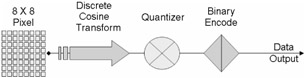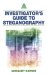# Embedding Methods

The technical challenges of data hiding are formidable. Any "holes" to fill with data in a host signal, either statistical or perceptual, are likely targets for removal by lossy signal compression. The key to successful data hiding is the finding of holes that are not suitable for exploitation by compression algorithms.

### Least-Significant Bit (LSB)

Least-significant bit is the substitution method of steganography where the rightmost bit in a binary notation is replaced with a bit from the embedded message. This method provides "security through obscurity," a technique that can be rendered useless if an attacker knows it is being used.

The two most important issues when using LSB substitution is the choice of the image and the choice of the format: 8-, 16-, or 24-bit, compressed or uncompressed.

The cover image first of all must seem casual, so it must be chosen between a set of subjects that can have a reason to be exchanged between the sender and the receiver. The image should have a lot of varying colors; it must be "noisy," so that the added noise is covered by the already present one. Wide solid-color areas show a lot of distortion when even a small amount of noise is added to them. Second, there is a problem with the file size, which involves the choice of the format. Unusually big files exchanged between two peers are likely to cause suspicion.

Most of the experts suggest using 8-bit grayscale images, because their palette is much less varied than the color one, so LSB insertion is very hard to detect by the human eye.

### Transform Techniques

There are three main types of transform techniques used when embedding a message in steganography: (1) discrete cosine transform (DCT), (2) discrete Fourier transform, and (3) wavelet transform.

#### Discrete Cosine Transform (DCT)

The discrete cosine transform, simply put, helps separate the image into parts of differing importance with respect to the image's visual quality. Discrete cosine transform-based image compression relies on two techniques to reduce the data required to represent the image:

1. Quantization of the image's DCT coefficients. Quantization is the process of reducing the number of possible values of a quantity, thereby reducing the number of bits needed to represent it.

2. Entropy coding of the quantized coefficients. Entropy coding is a technique for representing the quantized data as compactly as possible.

A simple example of quantization is the rounding of real numbers into integers. To represent a real number between 0 and 7 to some specified precision takes many bits. Rounding the number to the nearest integer gives a quantity that can be represented by just three bits.

For example, 2.765423 rounded to 3 takes up fewer bits. By doing this, we can reduce the number of possible values of the quantity, and along with it the number of bits needed to represent it, at the cost of losing information. A "finer" quantization allows for more values and loses less information.

In the JPEG image-compression standard, each cosine transform coefficient is quantized using a weight that depends on the frequencies for that coefficient. The coefficients in each 8 8 block are divided by a corresponding entry of an 8 8 quantization matrix, and the result is rounded to the nearest integer.

To shed some more light on the DCT, we will take a closer look at how JPEG compression works.

1. JPEG divides up the image into 8 8 pixel blocks, and then calculates the DCT of each block.

2. The DCT helps separate the image into parts (or spectral subbands) of differing importance (with respect to the image's visual quality). In other words, some parts of the image are more important to the overall picture than other parts.

3. A quantizer rounds off the DCT coefficients according to the quantization matrix. At this point it is important to reemphasize that there is a trade-off between image quality and the degree of quantization. A large quantization change can produce unacceptably large image distortion. On the opposite end, finer quantization leads to lower compression ratios. With this said, the question now is how to quantize the DCT coefficients most efficiently. Because of human eyesight's natural high-frequency roll-off, these high frequencies play a less important role than low frequencies. This lets JPEGs make larger modifications to the high frequencies with little noticeable image deterioration. If steganographic data is being loaded into the JPEG image, the loading occurs after this step.

4. This next step produces the "lossy" nature of a JPEG, but this also allows for large compression ratios.

5. JPEG's compression technique uses a variable length code and then writes the compressed data stream to the output file, with the commonly recognized .jpg suffix. During decompression, JPEG recovers the quantized DCT coefficients from the compressed data stream, takes the inverse, and displays the image (Figure 4.3).Figure 4.3

The JPEG encoding procedure divides an image into 8 8 blocks of pixels. Then they are run through a DCT and the resulting visual frequencies, high and low, are scaled to remove the ones that human viewers would not detect under normal conditions. If steganographic data is going to be loaded into the JPEG, it happens after this step. When this happens, the lowest-order bits of all nonzero frequency coefficients are replaced with the bits from the steganographic source file. These modified coefficients are then sent to the Huffmann coder, which changes color frequencies to a numeric value.

Here is an example showing how steganographic data is encoded:

• The steganographic encoding format (the format of data inserted into the lowest-order bits of the image) is as follows:

` + — — -+ — — — — — - — — -+ — — — — — — — — — — — — — — — —             | A | B B B… B | C C C C C C C C C C… + — — -+ — — — — — - — — -+ — — — — — — — — — — — — — — — — `

• "A" is 5 bits. It expresses the length (in bits) of field B. Order is most-significant bit first.

• "B" is some number of bits from 0 to 31. It expresses the length (in bytes) of the injection file. Order is again most-significant bit first. The range of values for "B" is 0 to 1 billion.

• "C" is the bits in the injection file. No ordering is implicit on the bit stream.

This format, by design, makes the steganographic content as inconspicuous as possible. But being inconspicuous is only part of the problem. The storage effectiveness for this technique is decent but not outstanding. Tests have shown that compressing the steganographic file before injecting the message does not greatly harm compression.

#### Discrete Fourier Transform

The discrete Fourier transform transforms a signal or image from the spatial domain to the frequency domain. Kun-Hung Lee, an engineering student at the University of Bridgeport, creates an excellent analogy for the discrete Fourier transform by comparing how sound frequencies are interpreted by the human ear.

##### Frequency Information as a Function of Time

An organ in our inner ears called the cochlea enables us to detect tonality in the sounds we hear. The cochlea is acoustically coupled to the eardrum by a series of three tiny bones. It consists of a spiral of tissue filled with liquid and thousands of tiny hairs. The hairs on the outside of the spiral are longer than the hairs on the inside of the spiral. Each hair is connected to a nerve that feeds into the auditory nerve bundle going to the brain. The longer hairs resonate with lower frequency sounds, and the shorter hairs with higher frequencies. Thus the cochlea serves to transform the air pressure signal experienced by the eardrum into frequency information that can be interpreted by the brain as tonality and texture. This way, we can tell the difference between adjacent notes on a piano, even if they are played equally loud. The Fourier transform is another mathematical technique for doing a similar thing: resolving any time-domain function into a frequency spectrum, much like a prism splitting light into a spectrum of colors. This analogy is not perfect, but it gets the basic idea across.

There is another transform technique that you may come across if you delve deeper into the mathematics of steganography called the wavelet transform, which is very similar in concept to the Fourier transform.

Spread-spectrum encoding is the method of hiding a small or narrow-band signal, a message, in a larger cover signal. The foundation of this process begins with a spread-spectrum encoder. The encoder works by modulating a narrowband signal over a carrier. The carrier signal is continually shifted using a noise generator and a secret key that makes the noise seem random. The message is embedded in the existing noise of the carrier signal, spreading the narrow signal over a wide area. This decreases the density of the hidden signal and makes it much more difficult to detect within the overall carrier signal.

Spread-spectrum encoding allows for very high data rates because messages can be compressed before being encoded in the carrier signal. Redundant data can also be added to the signal for error correction. Spread-spectrum is usually very robust because the addition of noise does not usually destroy the message. However, it is possible to remove the message with noise reduction filters, which would be used by the intended recipient to extract the message.

Spread-spectrum encoding is a very good method of steganography because of its difficulty to detect; if it is detected, it is usually more difficult to decipher because the attacker would also need the secret key used to encode the message.

This form of steganography occurs when one signal or sound becomes imperceptible to the observer because of the presence of another signal. This method also exploits the weaknesses of the human visual and auditory systems. A common example that almost everyone has seen is in spy films where someone is trying to communicate with someone else in a room they know is bugged. Usually the secret agent will turn up the stereo, run the shower, or do some other innocent (but noisy) task that allows a whispered conversation to take place without being heard.Investigators Guide to Steganography
ISBN: 0849324335
EAN: 2147483647
Year: 2003
Pages: 220

Similar book on Amazon